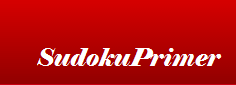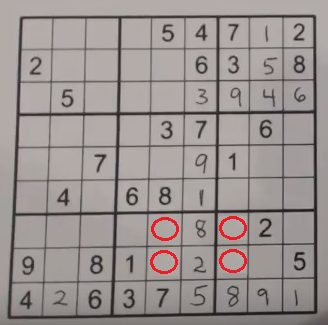﻿
Sudoku Primer & Complete ReferenceTool: Sudoku Ambiguity rule (Sudoku Unique Rectangles)

Next technique: solving single cells >>

Introduction
When Sudoku puzzles are created the designers make sure that there is one and only one solution to a given puzzle. This means that you can not solve it in more than one way. Every cell in the puzzle can only have one of the nine numbers in it. If a cell has a 5 in it, for example, the puzzle cannot be correctly solved with any other number in that cell.

One strategy for designing a puzzle is to selectively remove numbers and then try to solve the puzzle with the remaining numbers. During this process the designer must be careful to not remove two numbers that would cause an ambiguity (where those two numbers could be swapped and the puzzle could be completed two different ways, therefore having two solutions).

This is what is called the sudoku ambiguity rule. Many puzzle solvers call this situation sudoku Unique Rectangles, or sudoku UR for short.

Details
We can take advantage of this and use it to give us clues about where numbers can go. Look at the following puzzle. The four cells that are circled are a place where we can use the ambiguity rule.Puzzle #88 from Sudoku To Go volume 103 by Soap Opera Digest

Now let's look at this puzzle and see what is possible in these cells.Box eight (b8) has three empty cells and is missing 4, 6 and 9. With the obvious clues we have the 4 can fit in any empty cell. The 6 can only fit in c5 (because of the 6 in b5) and the 9 can only fit in r7 (because of the 9 in b7).

Now look at b9 - it has two sets of twins that can't be solved yet, but we know they are twins. There are 37 twins in r7c9 and r8c8 (because of the 3 and 7 already in c7) and therefore there are 46 twins in the other two empty cells r7c7 and r8c7. The 46 twins will help us find where the 4 fits in b8.

With that knowledge let's look at b8 again. Since the 6 can only fit in c5 we cannot have a 4 in c5 because we would then have 46 twins in both b8 and b9 in row 7 and row 8. If that were the case, either box (b8 or b9) could have the 4 above the 6 or the 4 below the 6 (and the opposite in the other box), which would give the puzzle two solutions.

Therefore, because of this rule, the 4 must fit in r7c4. See the image below for the correct placement of the 4 in b8.Skill Levels
This advanced technique is most useful on hard and diabolical puzzles. It could certainly be used on easier puzzles but there are probably easier techniques that will stand out ahead of this rule, since the ambiguity rule is harder to spot.

Next technique: solving single cells >>